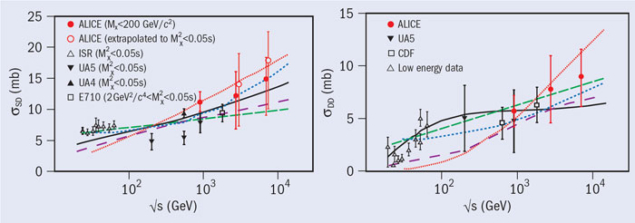# ALICE takes a first look at diffraction

6 November 2012Fig. 1. Schematic rapidity (y) distribution of outgoing particles in elastic (left), single-diffraction (middle) and double-diffraction (right) events, illustrating the typical rapidity-gap topology.

Diffractive processes represent more than 25% of the cross-section for inelastic proton–proton collisions at the LHC. So, it is only natural that the ALICE collaboration is interested in a field that started with optical phenomena but which today provides access to non-perturbative QCD processes, at the heart of ALICE’s scientific programme. There are also practical reasons that diffraction cannot be ignored, for instance, when normalizing data to specific event classes such as non-single diffractive (NSD) or inelastic (INEL), or when measuring precisely the proton–proton inelastic cross-section, which is used by ALICE as input for model calculations to determine the number of nucleon–nucleon binary collisions in heavy-ion collisions.

Diffractive reactions in particle physics are characterized by an exchange that has the quantum numbers of the vacuum – the pomeron – and leaves a “rapidity gap”, devoid of particles. Experimentally, there is no possibility to distinguish large rapidity gaps caused by pomeron exchange from those caused by other colour-neutral exchanges (e.g. secondary Reggeons). The ALICE collaboration therefore used the occurrence of large rapidity gaps as definition of diffraction (figure 1). Single Diffraction (SD) processes are those that have a large gap in rapidity from the leading proton, limited by the value of the diffracted mass MX < 200 GeV/c2 on the other side; other inelastic events are considered NSD events. Double diffraction (DD) processes are defined as NSD events with a gap in pseudorapidity, Δη > 3.Fig. 2. Single- and double-diffraction (Δη > 3) proton–proton and proton–antiproton cross-sections, left and right, respectively, as a function of centre-of-mass energy, compared with current models: Gotsman et al. (short, dot-dashed blue line), Goulianos (dashed green line), Kaidalov-Poghosyan (solid black line), Ostapchenko (long, dot-dashed pink line) and Ryskin et al. (dotted red line).

ALICE profited from particularly favourable circumstances in its study of diffraction: a detector sensitive to particles with low transverse-momentum, down to about 20 MeV/c; data taken with low luminosity so that corrections for event overlap in the same proton bunch-crossing are small; and above all, the presence in the collaboration of Martin Poghosyan, a developer of diffraction models and a former co-worker of the late Aleksei Kaidalov, which gave ALICE privileged access to the details of the Kaidalov-Poghosyan (KP) model.

The challenge was to study diffraction while being unable to observe either the non-diffracted proton or events in which the diffracted system escapes the acceptance of the detector. Nevertheless, the ALICE detectors cover a sufficient range in pseudorapidity (8.8 units, from –3.7 to 5.1, for collisions at the origin of the co-ordinate system) to have ample sensitivity to the SD and DD processes. Two independent observables were identified that are sensitive to diffraction: the ratio of the numbers of SD-like (activity on one side of the detector only) to NSD-like (activity on both sides of the detector) events; and the width distribution of the pseudorapidity gap for events of NSD type.

Obtaining the relative rates of diffractive processes from these two observables required the use of a model, so ALICE chose the KP model to estimate the fraction of unseen events. In practice, the diffracted-mass distribution is the sole relatively unknown parameter – the kinematics of diffractive collisions and the fragmentation of the diffracted system are known with sufficient precision. Experimental data and recent models that include higher-order pomeron terms show that the variation of the diffracted-mass distribution with centre-of-mass energy is slow, which gives confidence that extrapolation to LHC energies does not add a large uncertainty.

The sensitivity to models was studied by considering different models for the diffracted-mass distribution. The systematic error was obtained from extreme cases, varying the KP model by a factor of ±50% at the low-mass threshold and using the Donnachie-Landshoff model as the other limit. A van der Meer scan of the transverse profiles of the beams provided the luminosity L. The simulation needed to be adjusted using the KP model and the observed relative rate of diffraction to determine the acceptance and efficiency factor, A, in the measurement of the trigger rate, R(t), so that the inelastic cross-section, σINEL, could be determined, using R(t) = A × σINEL × L. Combining the relative rates of diffractive processes with inelastic cross-sections, ALICE obtained the SD and DD cross-sections at three centre-of-mass energies, √s = 0.9, 2.76 and 7 TeV, as shown in figure 2. (σINEL at √s = 0.9 TeV was not measured by ALICE, instead, σINEL = 52.5+2.0/–3.3 was used.)

This first measurement of SD and DD cross-sections at the LHC confirms that these processes evolve only slowly from the centre-of-mass energies of the Intersecting Storage Rings to 7 TeV at the LHC. The analysis by ALICE also shows the importance of including diffraction correctly to describe precisely the acceptance and efficiency of the detector for minimum bias triggers.